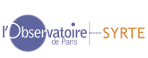BACK
Non tidal oceanic effect on nutation
NON-TIDAL OCEANIC DIURNAL AND SEMI-DIURNAL POLAR MOTION

At tidal diurnal and semi-diurnal periods, water heigh and currents present non-tidal variations, partly caused by atmospheric wind and pressure variations. The effect on diurnal and semi-diurnal polar motion can be derived from non tidal equatorial oceanic angular momentum (OAM) series, as in Brzezinski et al. (2004), Tab. 3 (prograde diurnal terms) and Tab. 4 (prograde + retrograde semi-diurnal terms). The estimated model reads

Δx = Σj pxcosj cos ξj+pxsinj sin ξj
Δy = Σj pycosj cos ξj + pysinj sin ξj

where ξj is the tidal argument, which is a integer linear combination of Delaunay arguments and of GMST + π : χj = a1 l + a2 l' + a3 D + a4 F + a5 Ω + a6 (GMST + π) ( a6 = 1 for diurnal terms, a6=2 for semi-diurnal terms)
```
Delaunay arguments
(IERS Conventions 2000, from Simon et al., 1994, Astron. Astrophys. 282, 663-683):

Mean anomaly of the Moon :
l = 134°.963 402 51 + 1 717 915 923.2178" t + 31".879 2 t2 + 0".051 635 t3 - 0".000 244 70 t4

Mean anomaly of the Sun  :
l'= 357°.529 109 18 +   129 596 581.0481" t - 0".553 2 t2 -  0".000 136 t3 - 0".000 011 49 t4

F = L -Ω  with L mean longitude of the Moon
F =  93°.272 090 62 + 1 739 527 262.8478" t - 12".751 2 t2 - 0".001 037 t3 + 0".000 004 17 t4

Mean elongation of the Moon from the Sun :
D = 297°.850 195 47 + 1 602 961 601.2090" t -  6".370 6 t2 + 0".006 593 t3 - 0".000 031 69 t4

Mean longitude of the ascending node of the Moon :
Ω = 125°.044 555 01  - 6 962 890.543 1" t + 7".472 2 t2 + 0".007 702 t3 - 0".000 059 39 t4

where t is measured un Julian Centuries of 36525 days of 86400 seconds of Dynamical Time since J2000.0.
```